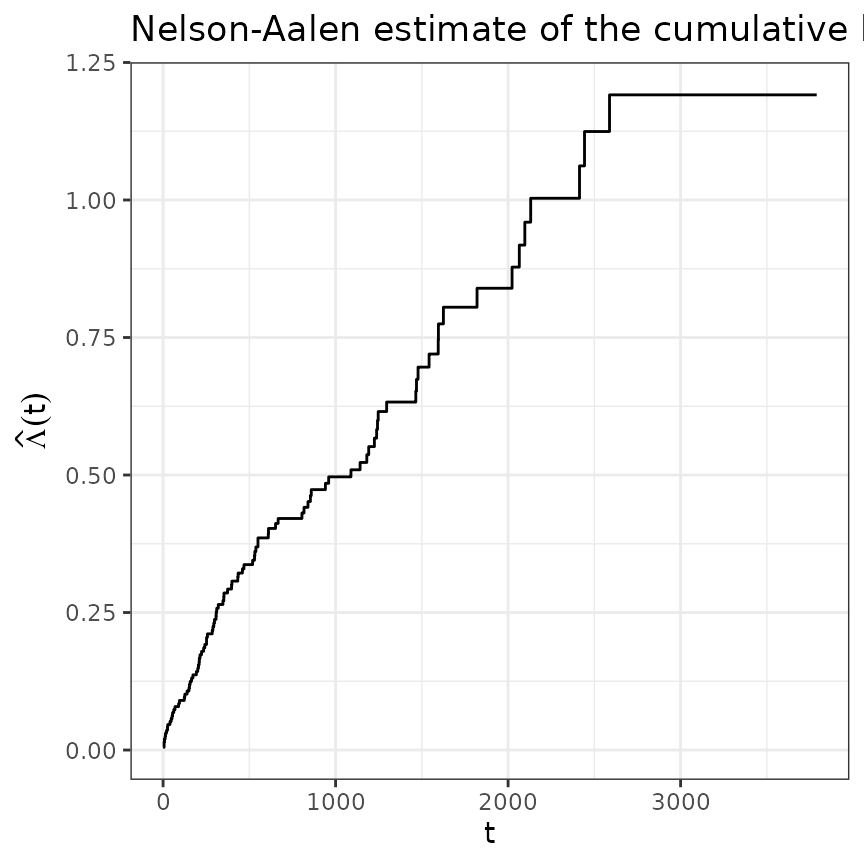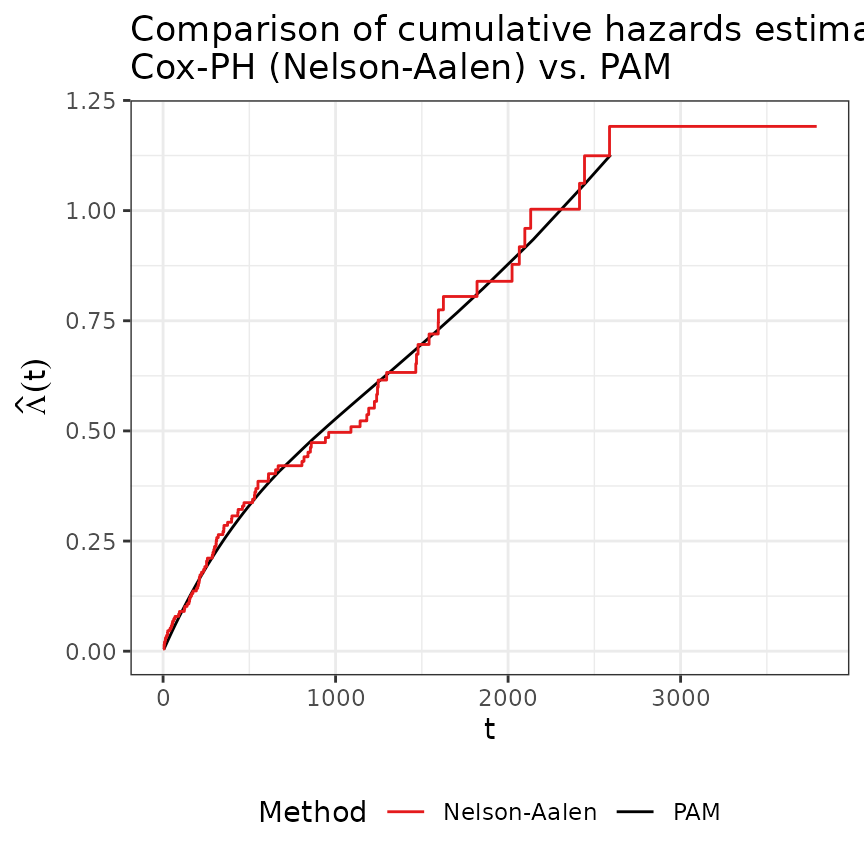library(ggplot2)
theme_set(theme_bw())
library(dplyr)
library(survival)
library(mgcv)
library(pammtools)
Set1 <- RColorBrewer::brewer.pal(9, "Set1")

Here we briefly demonstrate how to fit and visualize a simple baseline model using the pammtools package. We illustrate the procedure using a subset of the tumor data from the pammtools package:

data("tumor")
head(tumor)
## # A tibble: 6 × 9
##    days status charlson_score   age sex    transfusion complications metastases
##   <dbl>  <int>          <int> <int> <fct>  <fct>       <fct>         <fct>
## 1   579      0              2    58 female yes         no            yes
## 2  1192      0              2    52 male   no          yes           yes
## 3   308      1              2    74 female yes         no            yes
## 4    33      1              2    57 male   yes         yes           yes
## 5   397      1              2    30 female yes         no            yes
## 6  1219      0              2    66 female yes         no            yes
## # ℹ 1 more variable: resection <fct>
tumor <- tumor[1:200,]

The below graph depicts the estimated cumulative hazard using the Nelson-Aalen estimator:

base_df <- basehaz(coxph(Surv(days, status)~1, data = tumor)) %>%
rename(nelson_aalen = hazard)
ggplot(base_df, aes(x = time, y = nelson_aalen)) +
geom_stephazard() +
ylab(expression(hat(Lambda)(t))) + xlab("t") +
ggtitle("Nelson-Aalen estimate of the cumulative hazard")
## Warning: Unknown or uninitialised column: linewidth.
## Warning: Unknown or uninitialised column: size.## Data transformation

To fit a PAM, we first we need to bring the data in a suitable format (see vignette on data transformation).

# Use unique event times as interval break points
ped <- tumor %>% as_ped(Surv(days, status)~., id = "id")
head(ped[, 1:10])
##   id tstart tend interval    offset ped_status charlson_score age    sex
## 1  1      0    5    (0,5] 1.6094379          0              2  58 female
## 2  1      5    8    (5,8] 1.0986123          0              2  58 female
## 3  1      8   10   (8,10] 0.6931472          0              2  58 female
## 4  1     10   14  (10,14] 1.3862944          0              2  58 female
## 5  1     14   20  (14,20] 1.7917595          0              2  58 female
## 6  1     20   26  (20,26] 1.7917595          0              2  58 female
##   transfusion
## 1         yes
## 2         yes
## 3         yes
## 4         yes
## 5         yes
## 6         yes

## PAMs

PAMs estimate the baseline log-hazard rate semi-parametrically as a smooth, non-linear function evaluated at the end-points tend of the intervals defined for our model.

Note that the estimated log-hazard value at time-points tend gives the value of the log-hazard rate for the entire previous interval as PAMs estimate hazard rates that are constant in each interval.

Estimating the log hazard rate as a smooth function evaluated at tend - instead of using an unpenalized estimator without such a smoothness assumption - ensures that the hazard rate does not change too rapidly from interval to interval unless there is sufficient evidence for such changes in the data.

pam <- gam(ped_status ~ s(tend), data = ped, offset = offset, family = poisson())
summary(pam)
##
## Family: poisson
##
## Formula:
## ped_status ~ s(tend)
##
## Parametric coefficients:
##             Estimate Std. Error z value Pr(>|z|)
## (Intercept)  -7.4044     0.1115   -66.4   <2e-16 ***
## ---
## Signif. codes:  0 '***' 0.001 '**' 0.01 '*' 0.05 '.' 0.1 ' ' 1
##
## Approximate significance of smooth terms:
##           edf Ref.df Chi.sq p-value
## s(tend) 2.188   2.72  12.37 0.00703 **
## ---
## Signif. codes:  0 '***' 0.001 '**' 0.01 '*' 0.05 '.' 0.1 ' ' 1
##
## R-sq.(adj) =  -0.00673   Deviance explained = 1.34%
## UBRE = -0.91507  Scale est. = 1         n = 11619

### Graphical comparison

In the figure below we compare the previous baseline estimates of the Cox model with the PAM estimates.

Expand here for R-Code
# Create new data set with one row per unique interval
int_df <- make_newdata(ped, tend = unique(tend)) %>%

gg_baseline <- ggplot(int_df, aes(x = tend)) +
geom_line(aes(y = cumu_hazard, col = "PAM")) +
geom_stephazard(data = base_df, aes(x=time, y = nelson_aalen, col = "Nelson-Aalen")) +
scale_color_manual(
name   = "Method",
values = c("PAM" = "black", "Nelson-Aalen" = Set1)) +
theme(legend.position = "bottom") +
ylab(expression(hat(Lambda)(t))) + xlab("t") +
ggtitle(paste0("Comparison of cumulative hazards estimated by\n",
"Cox-PH (Nelson-Aalen) vs. PAM"))Both models are in good agreement.